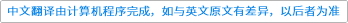Communications in Contemporary Mathematics ( IF 1.278 ) Pub Date : 2020-12-03 , DOI: 10.1142/s0219199720500789
Biagio Cassano

We determine the largest rate of exponential decay at infinity for non-trivial solutions to the Dirac equation being $𝒟n$ the massless Dirac operator in dimension $n≥2$ and $𝕍$ a (possibly non-Hermitian) matrix-valued perturbation such that $|𝕍(x)|∼|x|−𝜖$ at infinity, for $−∞<𝜖<1$. Also, we show that our results are sharp for $n∈{2,3}$, providing explicit examples of solutions that have the prescripted decay, in presence of a potential with the related behavior at infinity. As a consequence, we investigate the exponential decay at infinity for the eigenfunctions of the perturbed massive Dirac operator, and determine the sharpest possible decay in the case that $𝜖≤0$ and $n∈{2,3}$.down
wechat
bug